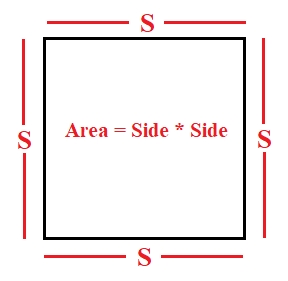# Program for Area Of Square in C++

We are given with a side of a rectangle and our task is to print the area of the square from that side.

Square is 2-D plain figure which have 4 sides and forms 4 angles of 90degree each and all the sides are of equal shape. In other words we can say that the square is a form of rectangle with equal sides.

Given below is representation of a square −The Area of square is Side x Side

## Example

Input: 6
Output: 36
As the side is 6 so the output is 6*6=36
Input: 12
Output: 144

## Algorithm

START
Step 1-> Declare a function int area(int side)
In function area
Declare a variable area and set it as side * side
Return area
End
Step 2 -> Declare a function int main()
In main Function
Declare a variable side and Set a Value
Call function area(side)
End
STOP

## Example

#include <iostream>
using namespace std;
//funcion to calculate area of square
int area(int side){
int area = side * side;
return area;
}
// Driver Code
int main(){
int side = 40;
cout <<"area of square is :"<<area(side);
return 0;
}

## Output

area of square is :1600